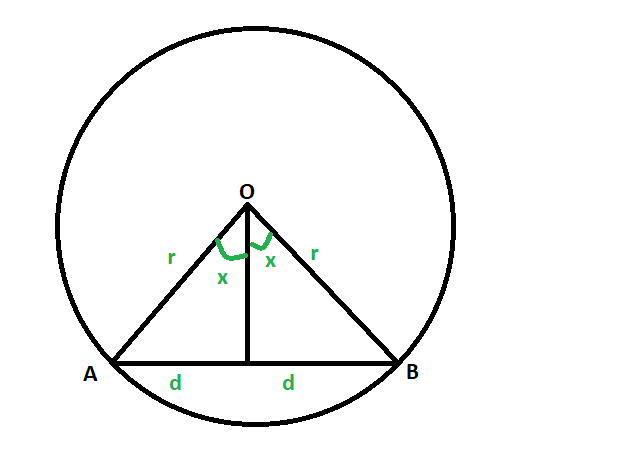# Length of the chord of the circle whose radius and the angle subtended at the center by the chord is given

Given a circle whose radius and the angle subtended at the centre by its chord is given. The task is to find the length of the chord.

Examples:

```Input: r = 4, x = 63
Output: 4.17809

Input:: r = 9, x = 71
Output:: 10.448
```Approach:

1. Let the circle has center at O and has radius r, and it’s chord be AB.
2. length of the chord be 2d, and the angle subtended by it on the center be 2x degrees.
3. As the perpendicular dropped at the chord bisects the chord so, the perpendicular also equally divides the subtended angle 2x in x degrees.
4. So, from the diagram,
d/r = sin(x*π/180)(here x deg is converted in radians)
5. So, d = rsin(x*π/180)
therefore, 2d = 2rsin(x*π/180)
6. So,Below is the implementation of the above approach:

## C++

 `// C++ program to find the length chord ` `// of the circle whose radius ` `// and the angle subtended at the centre ` `// is also given ` ` `  `#include ` `using` `namespace` `std; ` ` `  `// Function to find the length of the chord ` `void` `length_of_chord(``double` `r, ``double` `x) ` `{ ` `    ``cout << ``"The length of the chord"` `         ``<< ``" of the circle is "` `         ``<< 2 * r * ``sin``(x * (3.14 / 180)) ` `         ``<< endl; ` `} ` ` `  `// Driver code ` `int` `main() ` `{ ` `    ``double` `r = 4, x = 63; ` `    ``length_of_chord(r, x); ` `    ``return` `0; ` `} `

## Java

 `// Java program to find the length chord ` `// of the circle whose radius ` `// and the angle subtended at the centre ` `// is also given ` ` `  `class` `GFG  ` `{ ` ` `  `// Function to find the length of the chord ` `static` `void` `length_of_chord(``double` `r, ``double` `x) ` `{ ` `    ``System.out.println(``"The length of the chord"` `        ``+ ``" of the circle is "` `        ``+ ``2` `* r * Math.sin(x * (``3.14` `/ ``180``))); ` `} ` ` `  `// Driver code ` `public` `static` `void` `main(String[] args)  ` `{ ` `    ``double` `r = ``4``, x = ``63``; ` `    ``length_of_chord(r, x); ` `} ` `} ` ` `  `// This code contributed by Rajput-Ji `

## Python3

 `# Python3 program to find the length chord ` `# of the circle whose radius ` `# and the angle subtended at the centre ` `# is also given ` ` `  `import` `math as mt  ` ` `  `# Function to find the length of the chord ` `def` `length_of_chord(r, x): ` ` `  `    ``print``(``"The length of the chord"` `        ``,``" of the circle is "` `        ``,``2` `*` `r ``*` `mt.sin(x ``*` `(``3.14` `/` `180``))) ` ` `  ` `  `# Driver code ` `r ``=` `4` `x ``=` `63``; ` `length_of_chord(r, x) ` ` `  `# This code is contributed by mohit kumar `

## C#

 `// C# program to find the length chord  ` `// of the circle whose radius  ` `// and the angle subtended at the centre  ` `// is also given  ` `using` `System; ` ` `  `class` `GFG  ` `{ ` `     `  `    ``// Function to find the length of the chord  ` `    ``static` `void` `length_of_chord(``double` `r, ``double` `x)  ` `    ``{  ` `        ``Console.WriteLine(``"The length of the chord"` `+ ` `                        ``" of the circle is "` `+ ` `                        ``2 * r * Math.Sin(x * (3.14 / 180))); ` `                         `  `    ``}  ` ` `  `    ``// Driver code  ` `    ``public` `static` `void` `Main(String[] args)  ` `    ``{  ` `        ``double` `r = 4, x = 63;  ` `        ``length_of_chord(r, x); ` `    ``} ` `} ` ` `  `// This code is Contributed by Naman_Garg `

## PHP

 ` `

Output:

```The length of the chord of the circle is 7.12603
```

My Personal Notes arrow_drop_upCheck out this Author's contributed articles.

If you like GeeksforGeeks and would like to contribute, you can also write an article using contribute.geeksforgeeks.org or mail your article to contribute@geeksforgeeks.org. See your article appearing on the GeeksforGeeks main page and help other Geeks.

Please Improve this article if you find anything incorrect by clicking on the "Improve Article" button below.